Latest Banking jobs   »

# Quantitative Aptitude Quiz For FCI Phase I 2022- 21st September

Directions (1-5): In the following questions, calculate quantity I and quantity II, compare them and answer
(a) If quantity I > quantity II
(b) If quantity I < quantity II
(c) If quantity I ≥ quantity II
(d) if quantity I ≤ quantity II
(e) if quantity I = quantity II or no relation can be established

Q1. A shopkeeper marked up the price of an article 50% above cost price.
Quantity I, profit (in Rs): The shopkeeper gives two successive discounts of 10% and 15% on the article. The selling price of article is Rs 229.5.
Quantity II, discount (in Rs): Shopkeeper sold the article in Rs 253 and makes a profit of 15%.

Q2. Quantity I, interest earned: A person invest Rs 15000 at SI for 4 years at rate of 12.5% per annum.
Quantity II, interest earned: A person invests Rs 12500 at CI for 3 years at rate of 20%.

Q3. Quantity I, x: 7x²-23x+18=0
Quantity II, y:3y²-16y+21=0

Q4. A boat takes 10 hours to cover a distance of 800 km in downstream and 20 hours to cover the same distance in upstream.
Quantity I: Distance cover by the boat in downstream in 7 hours.
Quantity II: Distance cover by the boat in upstream in 13 hours.

Q5. Two persons A and C together can complete a piece of work in 10 days while B alone can complete the same piece of work in 24 days. A is twice as efficient as C.
Quantity I: Time taken by B and C together to complete the work.
Quantity II: Time taken by A and B together to complete the work.

Directions (6-10): The following questions are accompanied by two statements (I) and (II). You have to determine which statements(s) is/are sufficient/necessary to answer the questions.
(a) Statement (I) alone is sufficient to answer the question but statement (II) alone is not sufficient to answer the question.
(b) Statement (II) alone is sufficient to answer the question but statement (I) alone is not sufficient to answer the question.
(c) Both the statements taken together are necessary to answer the question, but neither of the statements alone is sufficient to answer the question.
(d) Either statement (I) or statement (II) by itself is sufficient to answer the question.
(e) Statements (I) and (II) taken together are not sufficient to answer the question.

Q6. What is the present age of Shivam.
I. Average of present ages of Dharam, Shivam and Abhishek is 20 years and ratio of their present ages is 5 : 3 : 4 respectively.
II. Abhishek is 5 year older than Shivam. Five years ago, age of Dharam was twice of age of Shivam.

Q7. What is the rate of interest?
I. Shubham invest an amount of Rs 384 at compound interest and after 3 years he got a sum
of Rs 750.
II. Amount becomes 3 times of sum if Shubham invests at simple interest for 8 years.

Q8. What is the distance?
I. A man reaches his office late by 12 min when his speed is 50 kmph, if he increases his speed by 22 kmph he will reach his office 10 min early.
II. A man covers half of distance at a speed of 30 kmph and remaining distance at speed of 60 kmph. In this whole journey he takes 1.5 hours.

Q9. In how much time Ankit can do the work alone?
I. Deepak and Ankit working together can compete a piece of work in 12 days and Deepak is half efficient than Ankit.
II. Dharam and Deepak working together can completes the work in 8 days while Ankit and Deepak working together can complete the same piece of work in 12 days.

Q10. What is the area of the rectangular park?
I. The breadth and length of the park are in the ratio of 2 : 3.
II. The perimeter of the rectangular park is 2000 m.

Directions (Q11-15): In the following questions, calculate quantity I and quantity II, compare them and answer
(a) If quantity I > quantity II
(b) If quantity I < quantity II
(c) If quantity I ≥ quantity II
(d) if quantity I ≤ quantity II
(e) if quantity I = quantity II or no relation can be established

Q11. Quantity I, Time (in months): A invests Rs 25,600 at CI at the rate of 12.5% per annum and after some time he got Rs 6800 as interest.
Quantity II, Time (in months): B borrows Rs 20,000 from bank at SI at the rate of 20% per annum. He settled up the amount by giving Rs 26,000 to bank after some time.

Q12. Quantity I, Time taken by Shivam to complete the work alone (in days): Dharam and Shivam working together can complete a piece of work in 7.5 days. If Dharam, Shivam and Anurag works together they complete the same work in 6 days. Efficiency of Shivam is 50% more than Anurag.
Quantity II: 23 days

Q13. Quantity I, x: 15x²-34x+15=0
Quantity II, y: 20y²-41y+20=0

Q14. A, B and C starts a business with investment of Rs 4000, Rs 5000, and Rs 10000, After 6 months, A invests Rs 2500 more while C withdraws all his money and after 8 months of business B invests Rs 2000 more. In the end of the year total profit earned by them is Rs 38200.
Quantity I: Total profit of A and B together.
Quantity II: Total profit of B and C together.

Q15. Quantity I: In how many ways letters of the word PHILIPS can be arranged.
Quantity II: in how many ways letters of the word TRAINING can be arranged.

Solutions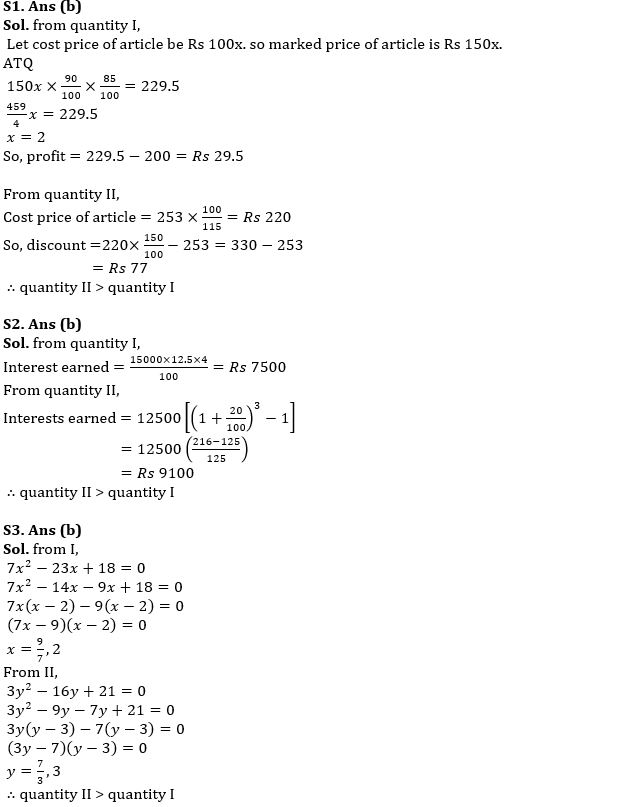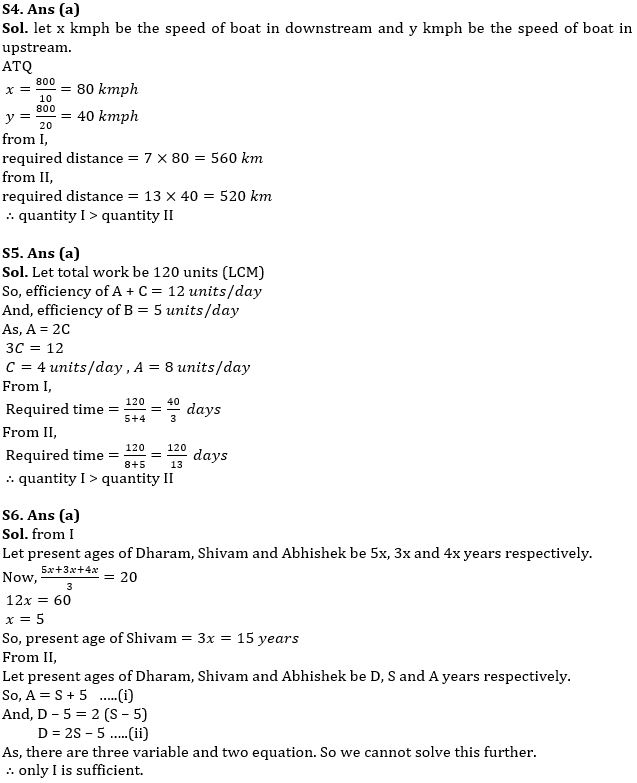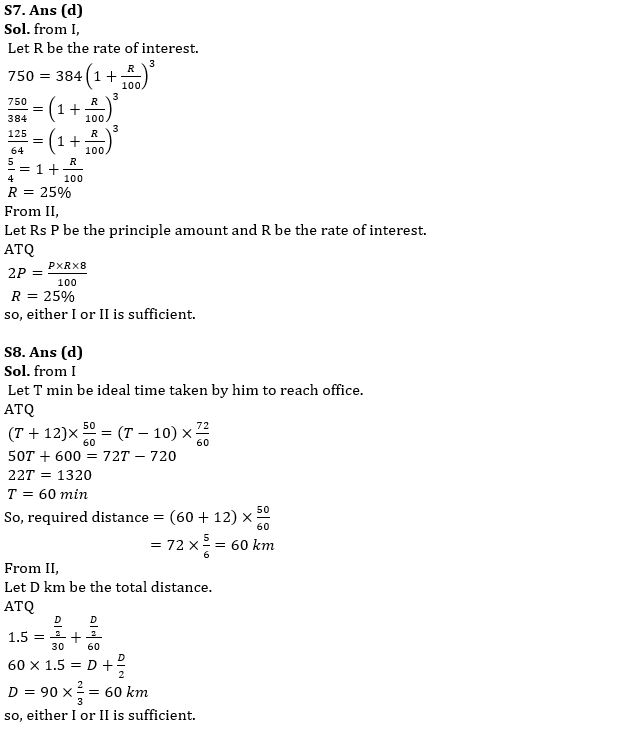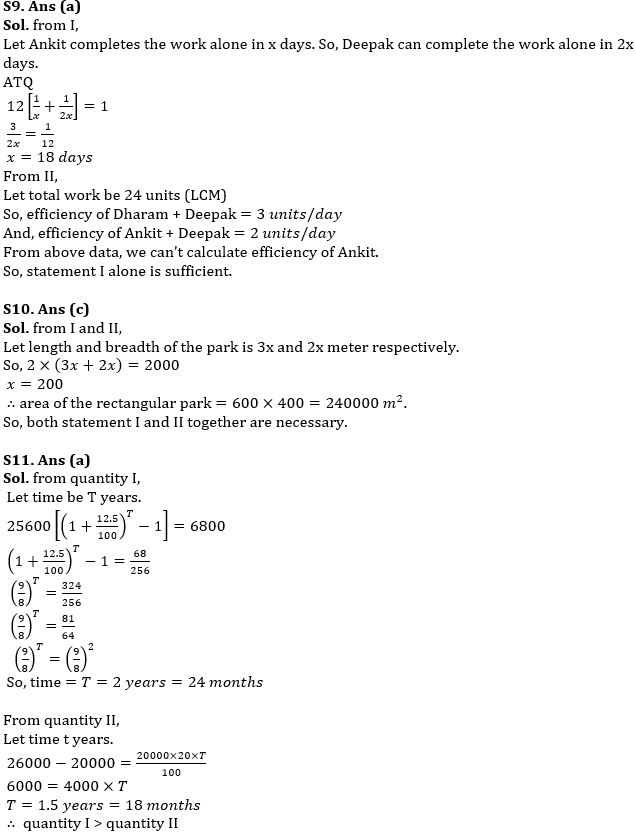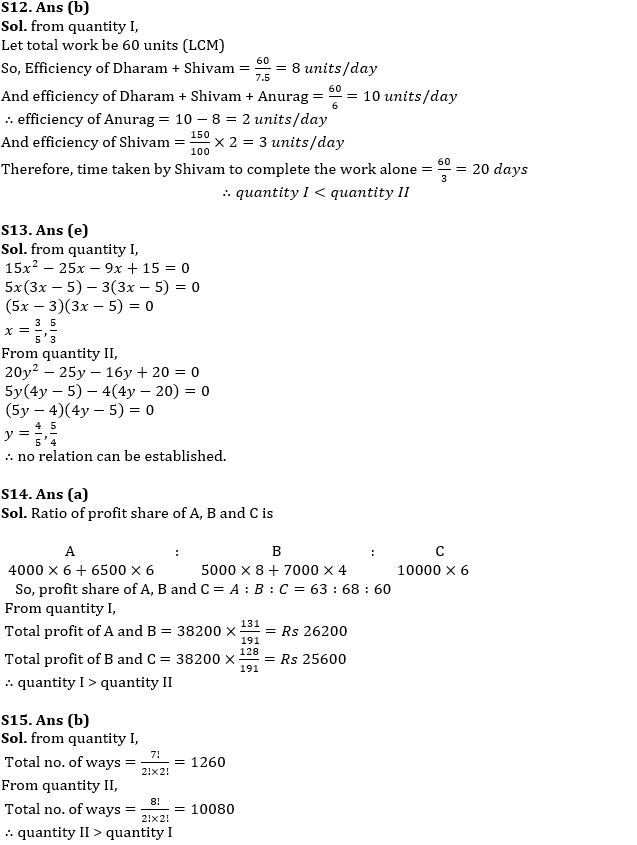#### Congratulations!Download Hindu Review of October 2021: Free PDF